# Analyzing the speed of spread of COVID-19 and publishing findings on dstack.ai

Subscribe to our newsletter and never miss any upcoming articles

As important and popular data science is, the very unfortunate situation around COVID-19 has raised even more interest in data science. Over the last couple of months, governments and individuals over the world are trying to collect data about COVID-19 and build models that can help predict the effect of the virus on our lives and economy, and understand how to save lives and fight the crisis.

Given that collaboration seems to be especially important for data science, I decided to write this article to demonstrate how one can use Python with some libraries to try to analyze the data about COVID-19, and possibly contribute to the joint effort by sharing their findings with the rest of the community — and of course, learning data science along the way.

• Use Python, pandas and plot.ly to analyze and visualize data. This may be interesting for beginner data scientists interested in learning Python in the context of analysing data.
• Use public data to analyse the influence of COVID-19.
• Use dstack.ai API for Python to publish analysis findings and share them with others.

Note, the analysis in this notebook serves education purposes and is not aimed at providing an accurate analysis. In case you find a mistake in the analysis or have a question related to the code or analysis results, please drop an email to andrey at dstack.ai.

In case you’re only learning Python for data science and don’t have an experience with Jupyter notebooks, we recommend you taking a look at it as it’s the most common way of working with data using Python.

When it comes to analysing data, the essential thing is good libraries at hand. In this tutorial, I’ll use the two most basic yet most important and popular libraries: pandas for data manipulation and plot.ly for making interactive and good-looking visualizations.

While pandas is the standard de facto for data manipulation in Python, there is actually more than one popular visualization libraries (e.g. Matplotlib and Bokeh to name a few). For the sake of simplicity, in this tutorial, I’ll use plotly.express which is a less-verbose wrapper around plotly.

Since I’d like to publish our data and visualizations on dstack.ai, I’ll use dstack Python library.

Another thing without which you cannot do data analysis is obviously data. In case your data is not clean, you’ll have to clean it yourself, e.g. using pandas. Cleaning data is another interesting topic in itself and is out of the scope of this tutorial. In our case, I’m going to use the data on confirmed cases of COVID-19 compiled from various sources and updated by John Hopkins University.

As you’ll see in the output of the next cell, the data I’ll be using provides the information on new confirmed cases of COVID-19 per province/state, country, and particular date. Here’s a little code that lets you load the data from a URL into a pandas datafame, and output a preview:

``````url = "https://data.humdata.org/hxlproxy/api/data-preview.csv?url=https%3A%2F%2Fraw.githubusercontent.com%2FCSSEGISandData%2FCOVID-19%2Fmaster%2Fcsse_covid_19_data%2Fcsse_covid_19_time_series%2Ftime_series_19-covid-Confirmed.csv&filename=time_series_2019-ncov-Confirmed.csv"
df = pd.read_csv(url) # returns a "pandas dataframe"
df.head() # this function displays the first 5 rows of the dataframe; it helps check the format of the data and make sure that everything is correct
view raw
``````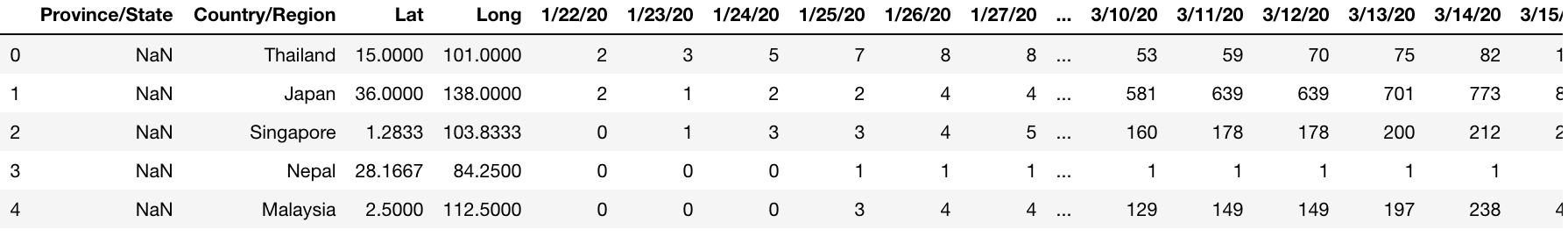One particular thing that may be of our interest is how the situation around COVID-19 is changing over the last two days for every country. To get this data, we’ll use pandas’s API to manipulate the data: e.g. drop unnecessary columns. In the code below, we’ll drop all columns except the country and the two last columns which are the confirmed cases for the last two days:

``````cols = [df.columns] + list(df.columns[-2:]) # this and below are a few of the ways how you can manipulate a dataframe using pandas
# country + two recent days (very simple, ignore week day, etc)
last_2_days = df[df["Province/State"].isnull()][cols].copy()
last_2_days # as you might've noticed above, the value of the expression in the end of the code cell is displayed in the output
view raw
``````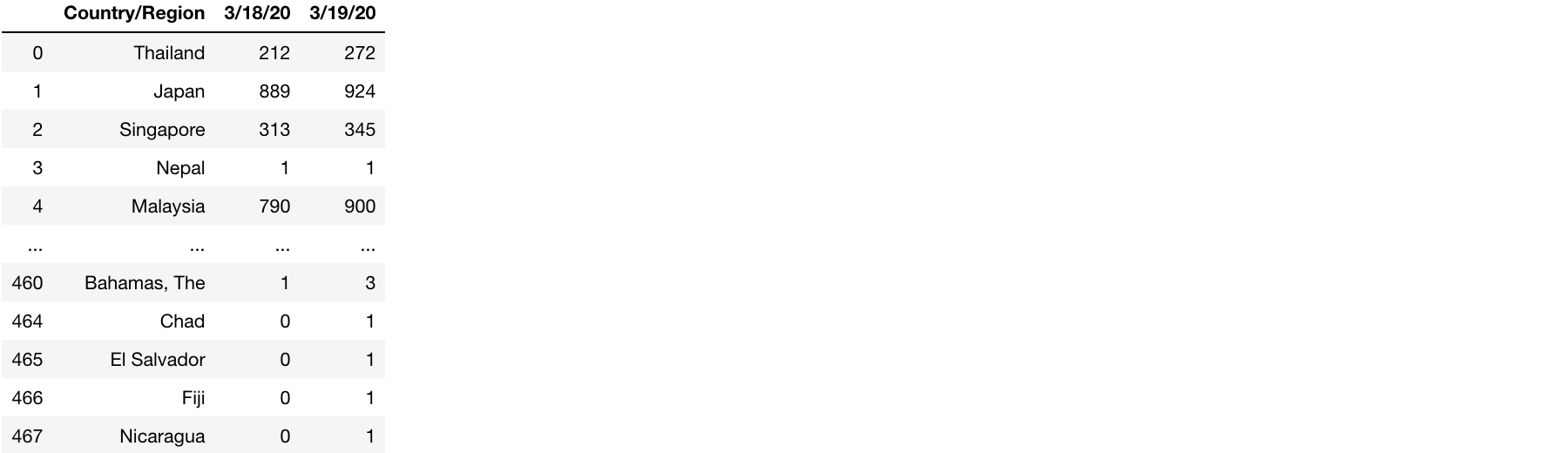One reason why the data of two following days can be interesting is that it lets you easily calculate the increase in new cases. The below code transforms the dataframe that we obtained earlier by extending it with two new columns: increase in absolute number of new cases, and increase in % of new cases:

``````d1 = last_2_days.columns[-1]
d2 = last_2_days.columns[-2]

last_2_days["delta"] = last_2_days[d1] - last_2_days[d2]
last_2_days["delta%"] = last_2_days["delta"] / last_2_days[d2]
last_2_days # displaying the resulting dataframe
``````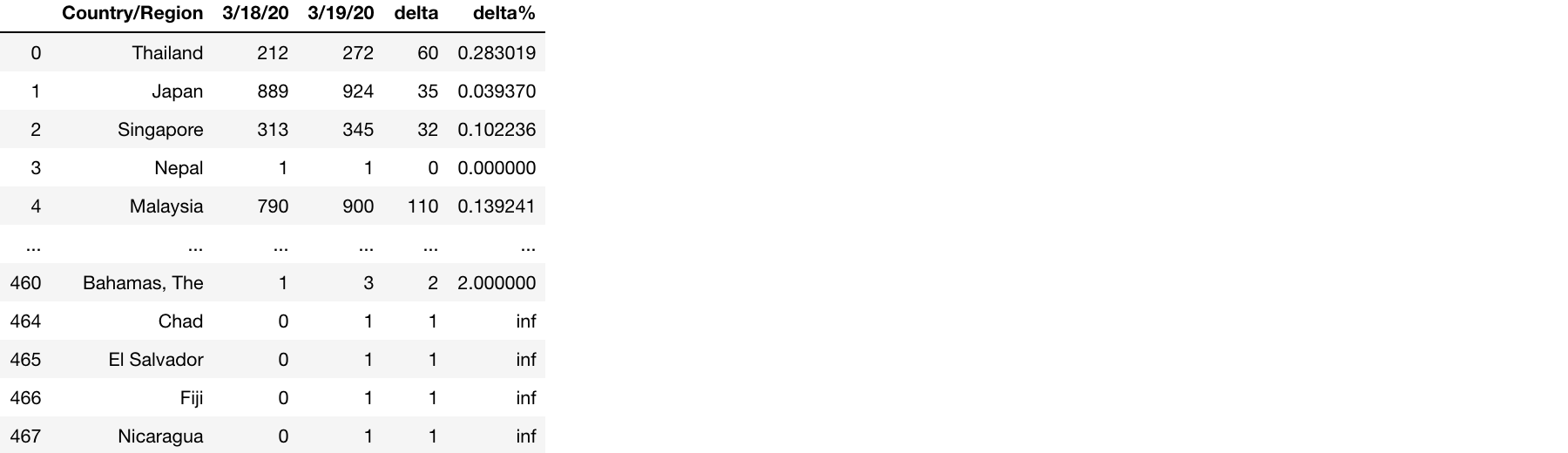Now, imagine you’d like to publish the resulting data online and share with other people. You can do that by using the dstack package. This package provides an API to publish both pandas dataframes and plotly visualizations (among other visualization libraries).

A nice thing about dstack is that it lets you publish data and visualizations and keep them interactive: e.g. the user may change parameters and see the data corresponding to chosen parameters.

Once the data is published to dstack.ai, it can be accessed via a link. Other people can access it and comment.

In order to publish pandas dataframes or plotly’s figures fro myour code, you have to create a dstack “frame” and specify the name of the “stack”. This “stack” can be later access via a URL, e.g. `https://dstack.ai/<user>/<stack>`. Every “stack” may have many “frames”. The “frames” are basically revisions of your published data. Each “stack” points to its “head frame” — the latest version of the published data. A “frame” includes a list of “attachments”. Every “attachment” can be a visualization or a dataframe and may have own parameters associated with it.

Don’t be afraid of too many concepts. It’s actually a very simple and very powerful abstraction that helps simplify managing data analysis results. To better understand this concept of stacks, frames and attachments, let’s see at our simple example.

1. Below, we create a frame and give it a stack name `covid19/speed`. This means that this frame will be published to a stack that can be accessed via `https://dstack.ai/<user>/covid19/speed`.

Note, the current user is configured via the `_dstack_` command line utility.

``````dstack config --token <token> --user <user>
``````

2. For both, increase in absolute numbers and increase in %, we’ll publish a separate dataframe so the user accessing the published data can switch between two tables. Each pandas dataframe is committed as a separate “attachment” along with a description and corresponding “attachment parameters”:

3. Push the “frame” to send all “attachments”

``````min_cases = 50
# create frame and set stack name
top_speed_frame = create_frame("covid19/speed")
# top countries
sort_by_cols = ["delta", "delta%"]
for col in sort_by_cols:
# commit attachment
top_speed_frame.commit(top, f"Top 50 countries with the fastest growing number of confirmed Covid-19 cases (at least {min_cases})", {"Sort by": col})

top_speed_frame.push()
``````

You can see the published stack at dstack.ai/cheptsov/covid19/speed.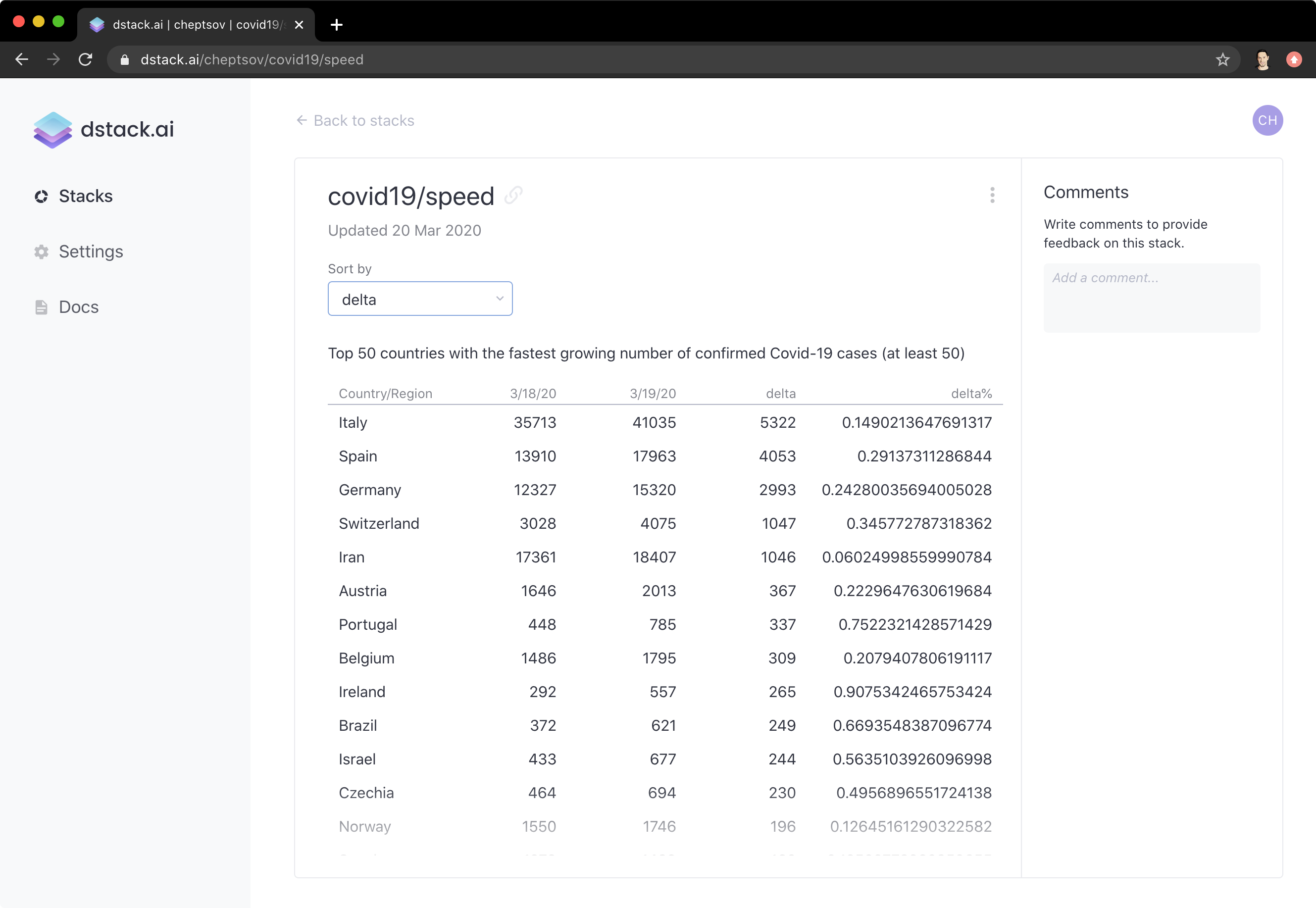Now let’s try to visualize some data, e.g. new cases for a given country over time. This exercise is great not only because it shows how to plot data, but also shows us more ways of manipulating data.

In order to plot our data on new cases over time, we’ll need to slightly change the format of the data — transpose the data, to make dates, dataframe rows instead of dataframe columns:

``````cdf = df[(df["Country/Region"]=="Italy") & (df["Province/State"].isnull())][df.columns[4:]].T
cdf = cdf.rename(columns={cdf.columns:"confirmed"}) # set the name of the new column resulted as a transposition of date dataframe columns
``````

After we have our data prepared, we can plot new cases. We’ll plot it as a line chart where the x axis is dates and the y axis is new cases:

``````fig = px.line(cdf, x=cdf.index, y="confirmed")
fig.show() # displays the `plotly`'s figure
``````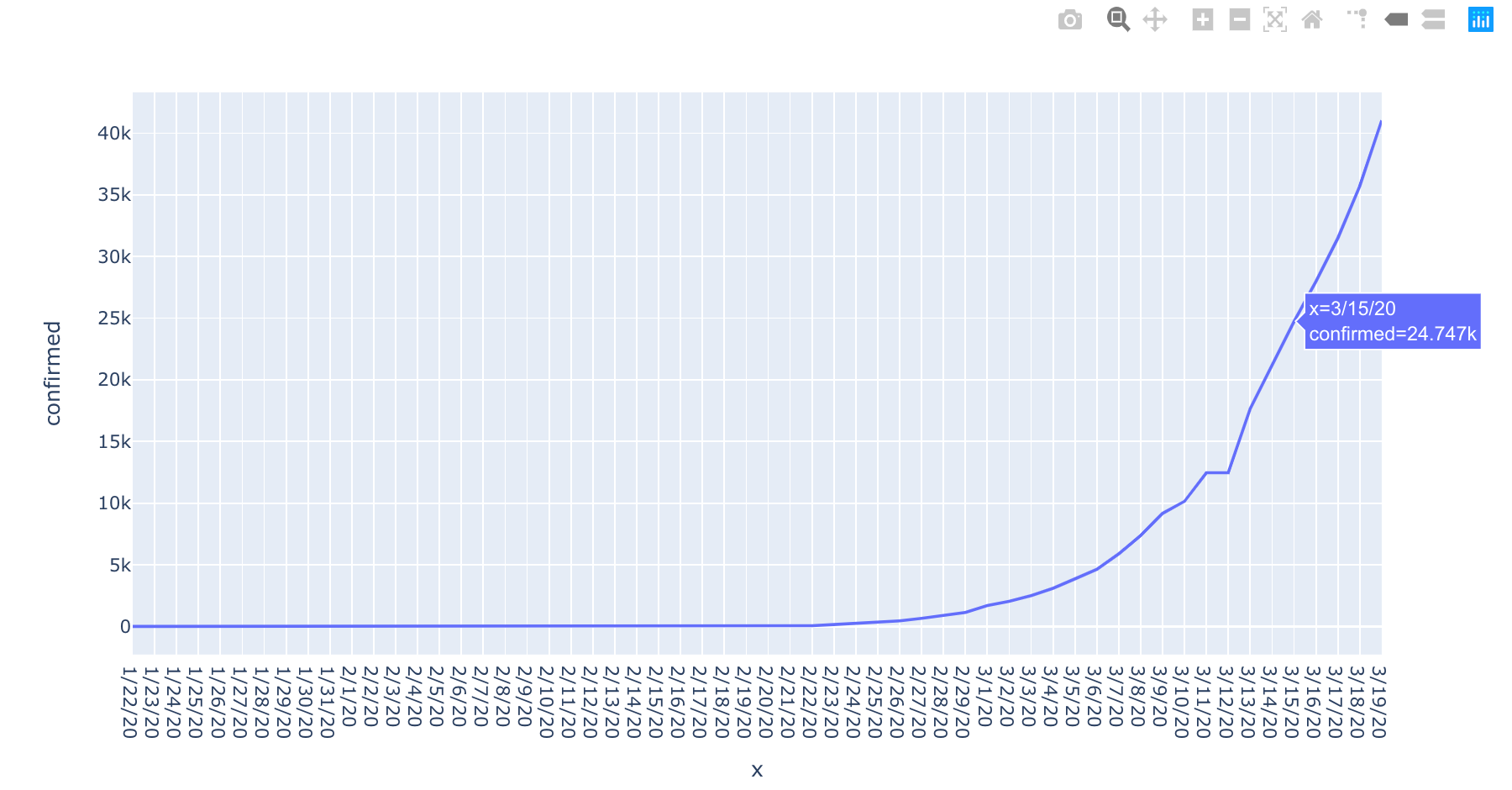Let’s now try to do something more advanced. How about visualizing increases in new cases over time?

To do that, we’ll need to do another manipulation with our dataframe. We’ll make a new dataframe by subtracting the number of new cases for the day by the number of new cases from the previous day. Here’s how it’s done using the panda’s API:

``````delta = (cdf.shift(-1) - cdf)
delta.tail() # display the last 5 rows of the dataframe to make sure the operation was correct
``````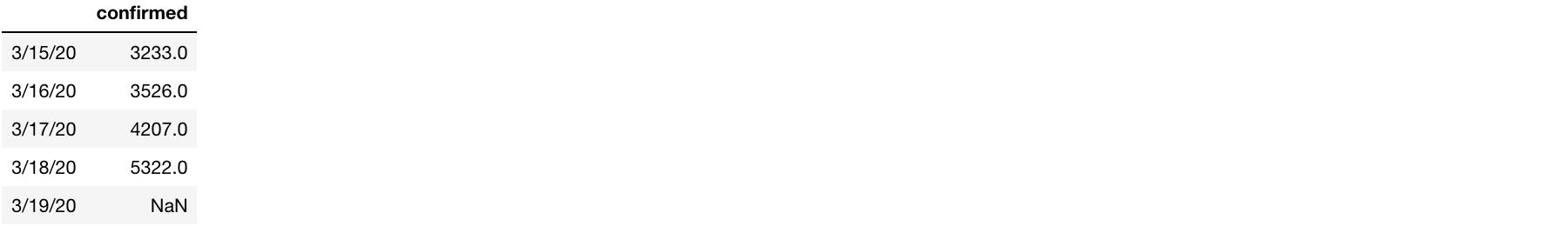Now the data is ready for plotting — the same way we did it above — with the difference that instead of the absolute number of cases, we display the increase, also in absolute numbers:

``````fig = px.line(delta, x=delta.index, y="confirmed")
fig.show()
``````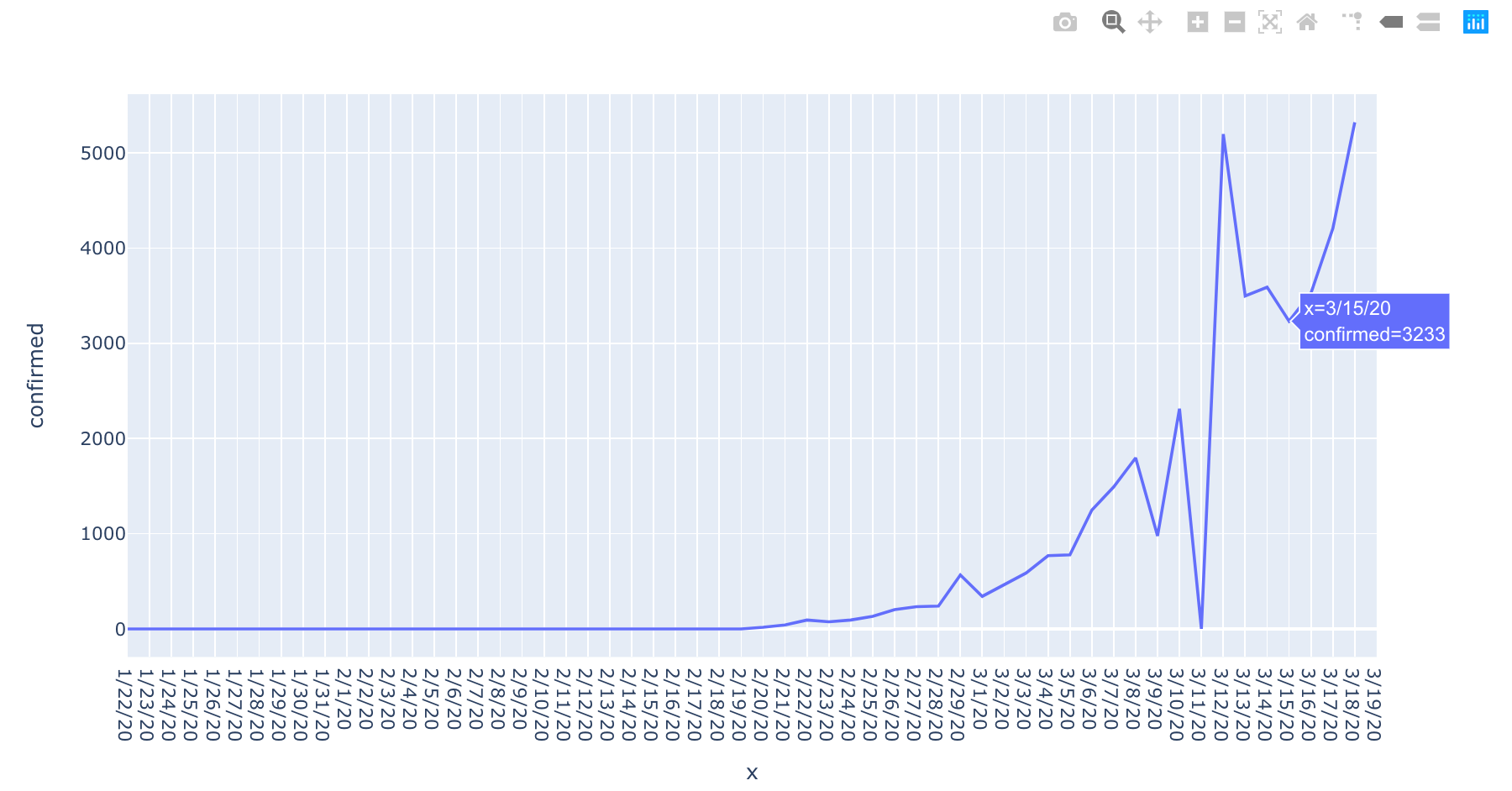By now, most of our coding above was simple, even though it may feel cryptic to you if you’re only getting familiar with pandas. Now, we’ll do a more advanced thing, we’ll generalize our code that manipulates data and makes plots by moving it to a function.

The function below returns three plotly figures: absolute confirmed cases, absolute increase, and increase in percent:

``````def plots_by_country(country):
cdf = df[(df["Country/Region"]==country) & (df["Province/State"].isnull())][df.columns[4:]].T
cdf = cdf.rename(columns={cdf.columns:"confirmed"})
cfig = px.line(cdf, x=cdf.index, y="confirmed")
delta = (cdf.shift(-1) - cdf).rename(columns={"confirmed": "confirmed per day"})
cdfig = px.line(delta, x=cdf.index, y="confirmed per day")
delta_p = ((cdf.shift(-1) - cdf) / cdf.shift(-1)).rename(columns={"confirmed": "confirmed per day %"})
cdpfig = px.line(delta_p, x=cdf.index, y="confirmed per day %")
return (cfig, cdfig, cdpfig)
``````

To test out function, let’s call it for Australia and display all three resulting plots one by one:

``````(fig1, fig2, fig3) = plots_by_country("Austria")
fig1.show()
fig2.show()
fig3.show()
``````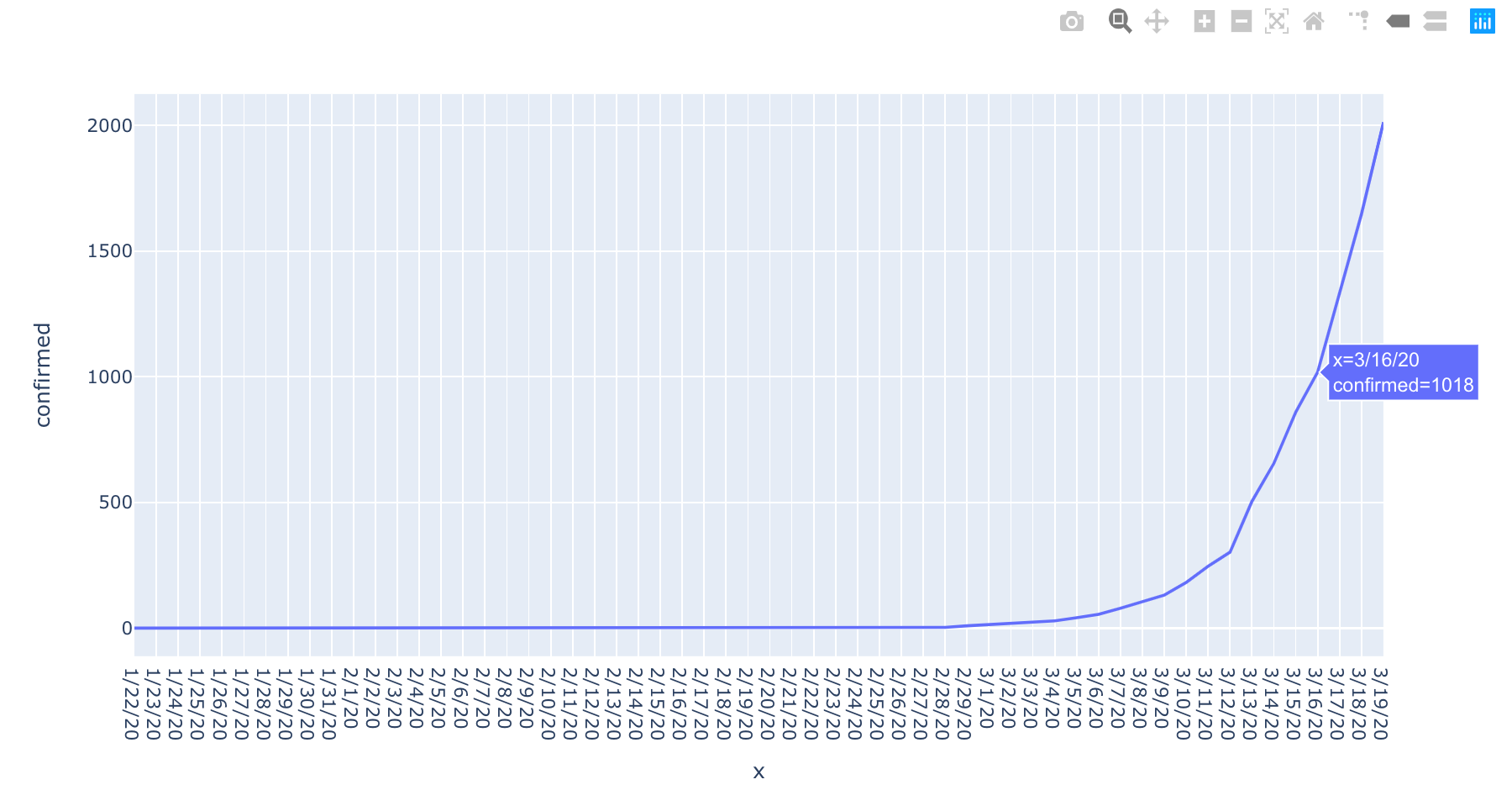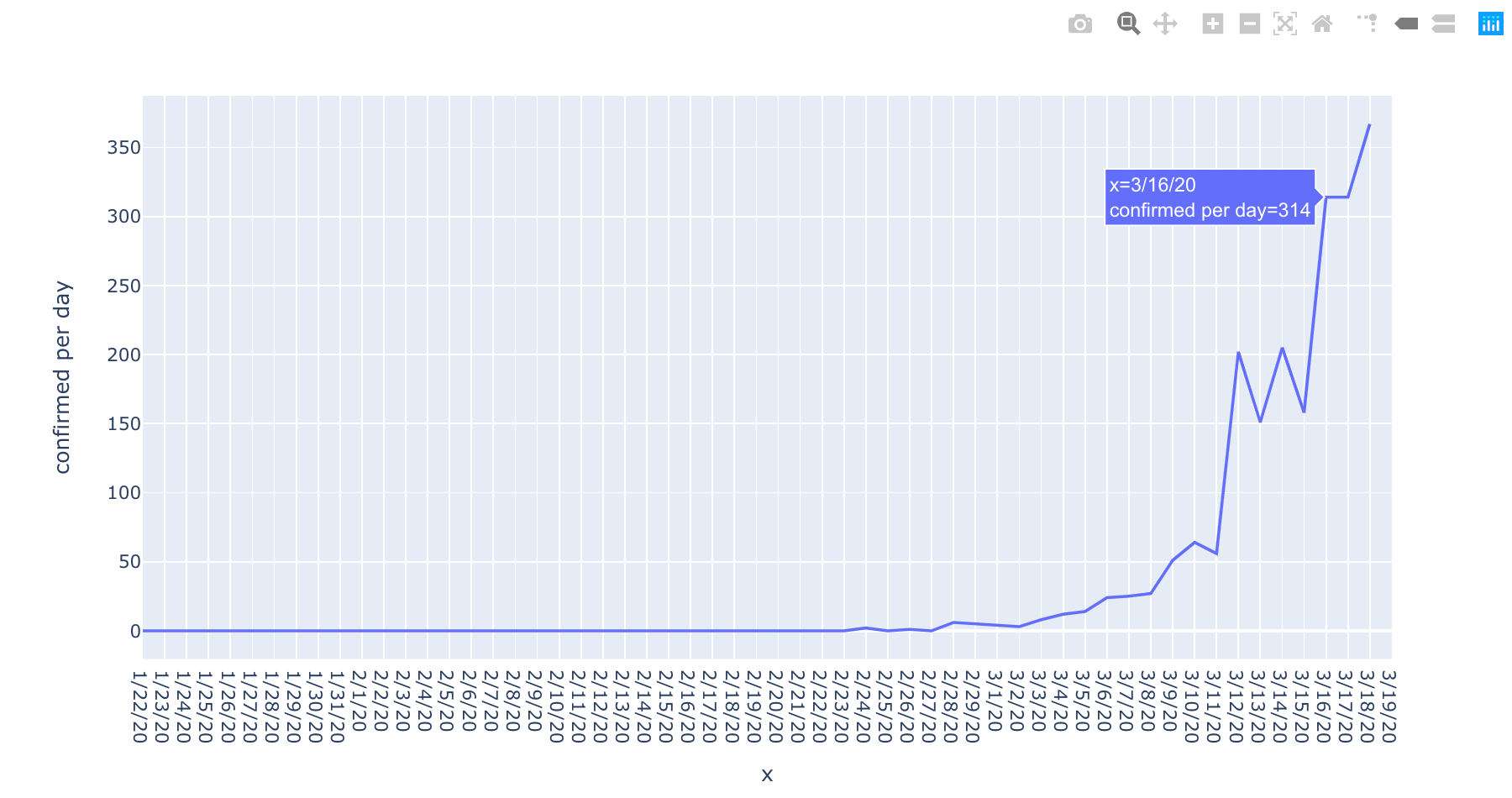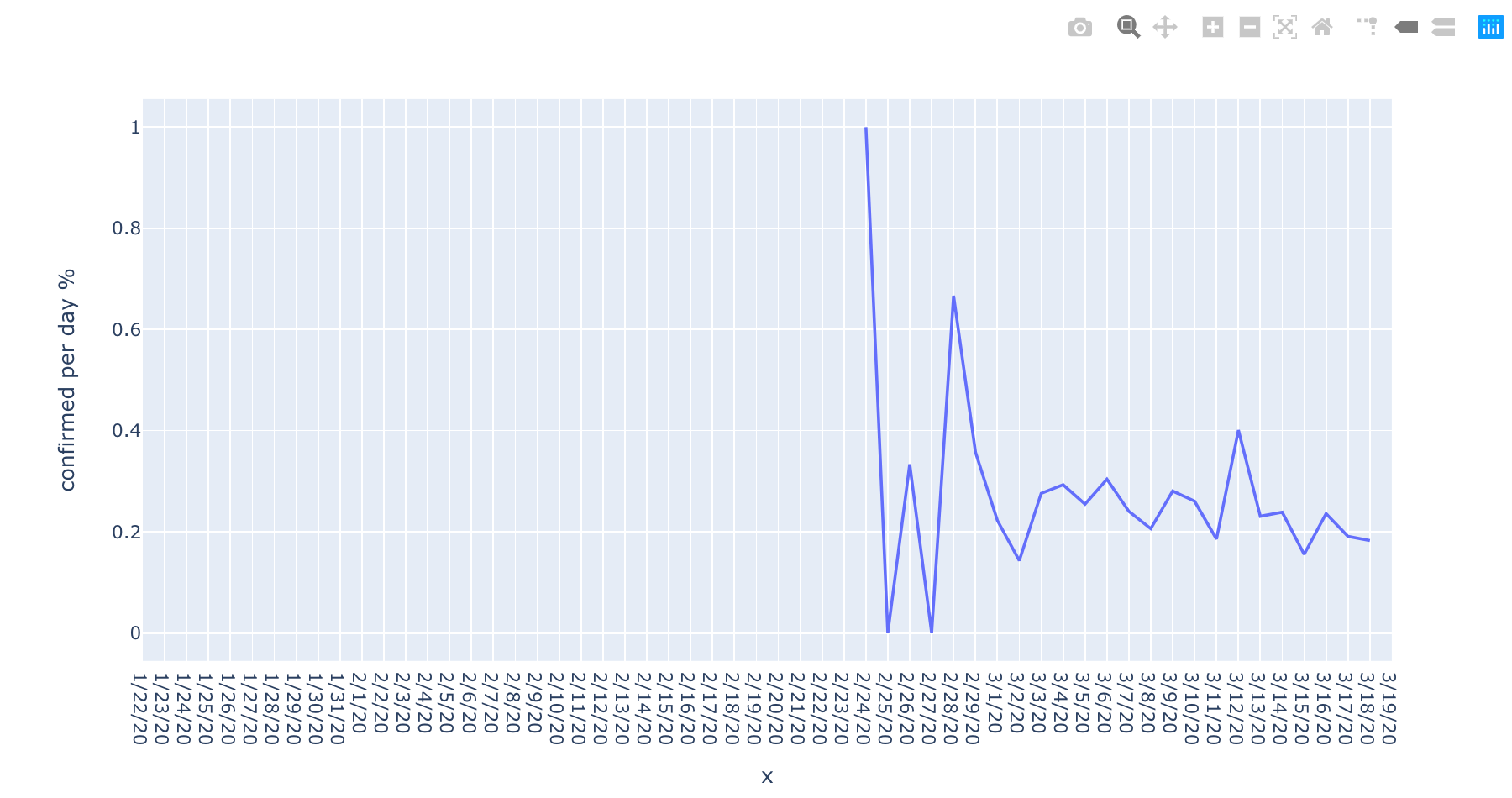Now, let’s use our function to call it on every country of the top 30 by new cases to publish all visualizations in one “stack”. This exercise will showcase how one single “stack” can be used to organize an interactive dashboard with multiple parameters. Here we go:

``````# get top 30 countries by the number of new cases on the last day

# create a frame and iterate over the top countries to commit three plots for every country: new absolute cases, increase in absolute numbers, and increase in percent
frame = create_frame("covid19/speed_by_country")
for c in countries["Country/Region"].tolist():
print(c)
(fig1, fig2, fig3) = plots_by_country(c)
frame.commit(fig1, f"Confirmed cases in {c}", {"Country": c, "Chart": "All cases"})
frame.commit(fig2, f"New confirmed cases in {c}", {"Country": c, "Chart": "New cases"})
frame.commit(fig3, f"New confirmed cases in {c} in %", {"Country": c, "Chart": "New cases (%)"})

frame.push()
``````

The published stack can be seen at dstack.ai/cheptsov/covid19/speed_by_country.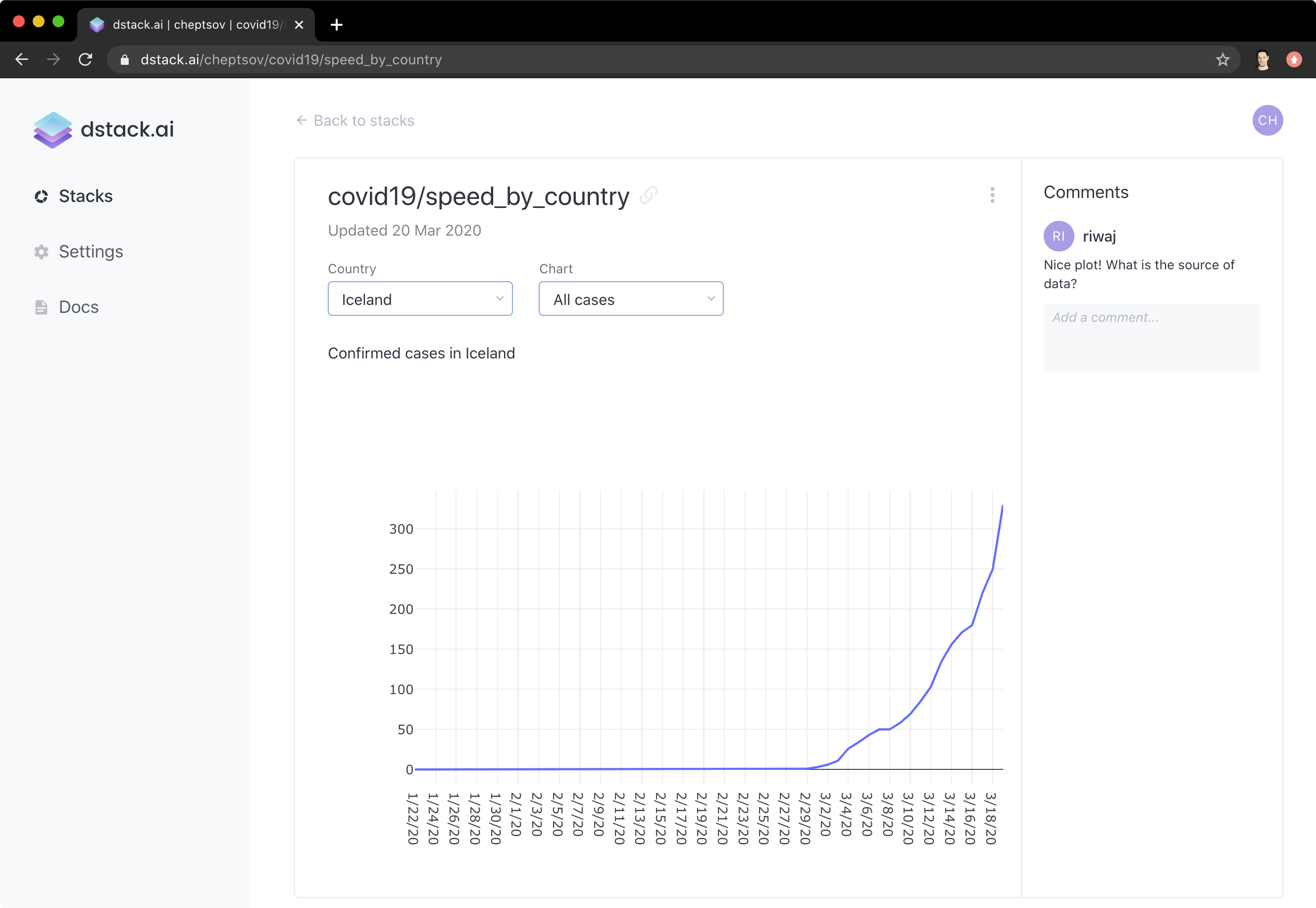To do another, a bit more comprehensive exercise on data manipulation, plotting, and also publishing, let’s try to visualize a similar data, but this time in addition to individual visualizations per country, also include a visualization with all countries together in one chart.

While doing this exercise, we’ll see one more interesting way of manipulating data. Let’s create a dataframe with absolute numbers of new cases for Italy for every day:

``````# filter Italy, transpose date dataframe columns into dataframe rows
t1 = df[(df["Country/Region"]=="Italy") & (df["Province/State"].isnull())][df.columns[4:]].T
# set the new column name
t1 = t1.rename(columns={t1.columns:"confirmed"})
# make the dataframe's index a regular column; we'll later need it to highlight each country with its own color
t1.reset_index()
t1["Country/Region"] = "Italy"
t1.tail() # display the last 5 rows to make sure everything is correct
``````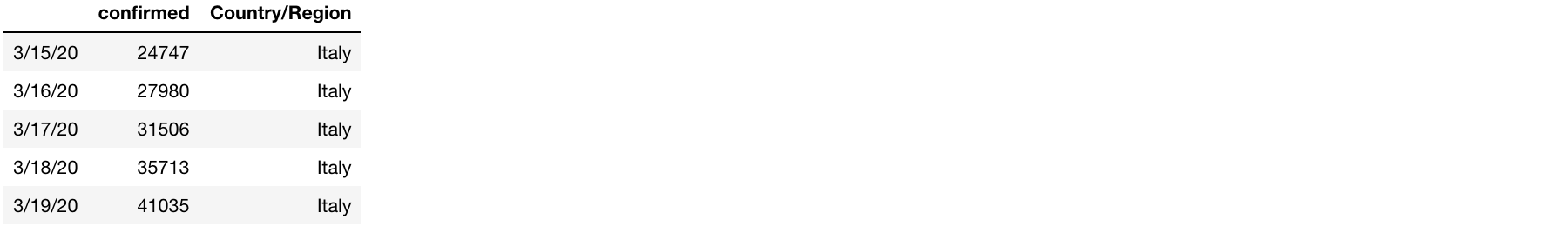Let’s generalize the code from above to make it work for a given country and also include increase in absolute numbers and in percent:

``````# this function return three dataframes: absolute new cases, absolute increase, percent increase
def country_df(country):
cdf = df[(df["Country/Region"]==country) & (df["Province/State"].isnull())][df.columns[4:]].T
cdf = cdf.rename(columns={cdf.columns:"confirmed"})
delta = (cdf.shift(-1) - cdf).rename(columns={"confirmed": "confirmed per day"})
delta.reset_index()
delta["Country/Region"] = country
delta_p = ((cdf.shift(-1) - cdf) / cdf.shift(-1)).rename(columns={"confirmed": "confirmed per day %"})
delta_p.reset_index()
delta_p["Country/Region"] = country
cdf.reset_index()
cdf["Country/Region"] = country
return (cdf, delta, delta_p)
``````

Now, let’s make a list of the top countries by the absolute number of new cases on the last day, and then make three list of dataframes for all countries: absolute new cases, absolute increase, percent increase.

``````# top 10 countries by last day absolute

# make a single lists of dataframes for all countries
top = []
top_delta = []
top_delta_p = []
for c in top10["Country/Region"].tolist():
(x, y, z) = country_df(c)
top.append(x)
top_delta.append(y)
top_delta_p.append(z)

test = pd.concat(top) # make a pandas dataframe out for the new cases
# plot the resulted dataframe of new cases to make sure everything is correct
px.line(test, x=test.index, y="confirmed", color='Country/Region').show()
``````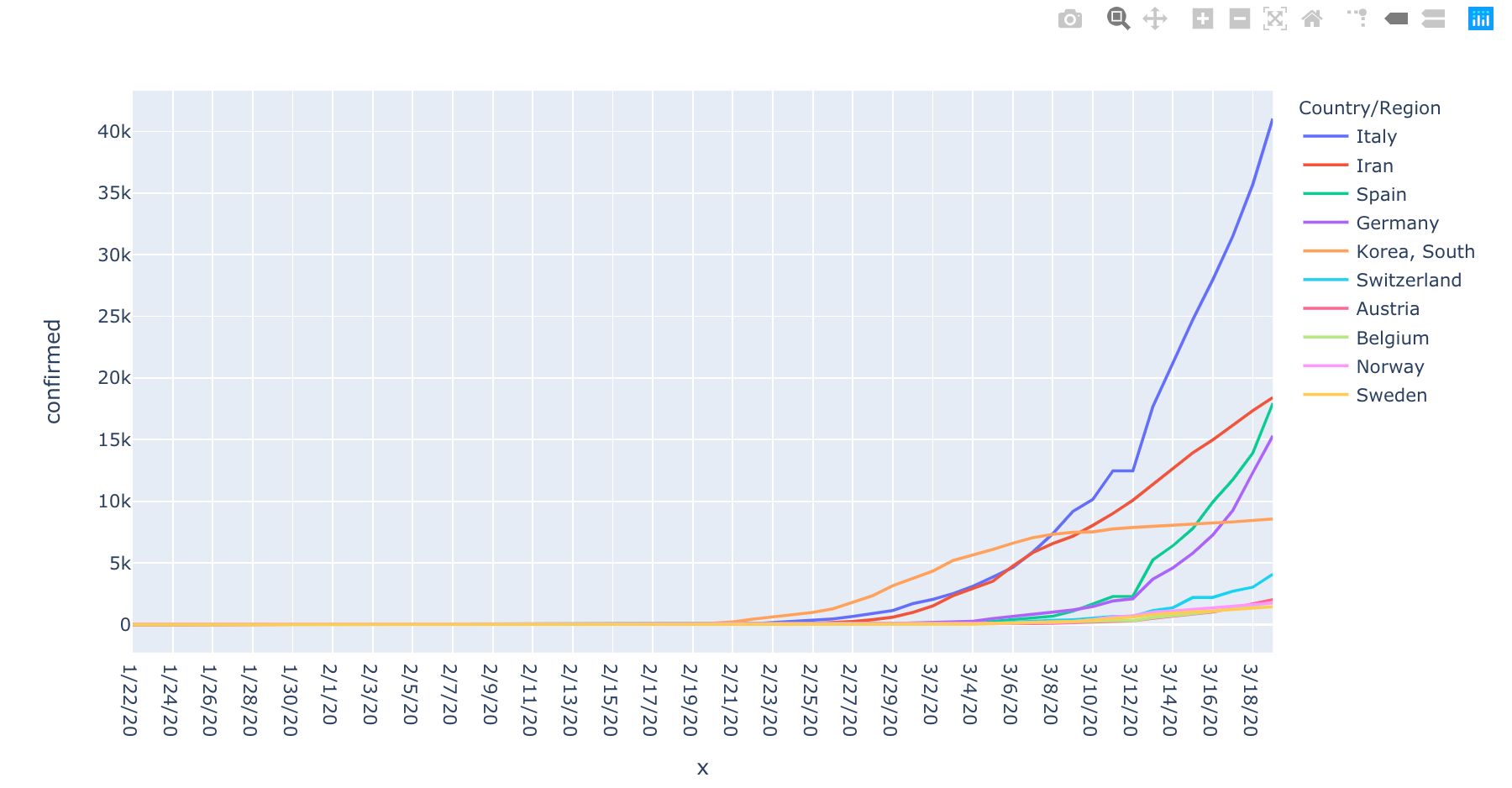Finally it’s time to put it all together, and use our function to make visualizations:

• Number of all cases over time for all 10 top countries
• Number of new cases over time for all 10 top countries
• Percent of increase over time for all 10 top countries
• Number of all cases over time for every of 30 top countries
• Number of new cases over time for every of 30 top countries
• Percent of increase over time for every of 30 top countries

Here’s the code that does it

``````frame = create_frame("covid19/speed_by_country_all")

top10df = pd.concat(top)
fig = px.line(top10df, x=top10df.index, y="confirmed", color='Country/Region')
frame.commit(fig, "Confirmed cases in top 10 countries", {"Country": "Top 10", "Chart": "All cases"})

top10df_delta = pd.concat(top_delta)
fig = px.line(top10df_delta, x=top10df_delta.index, y="confirmed per day", color='Country/Region')
frame.commit(fig, "New confirmed cases in top 10 countries", {"Country": "Top 10", "Chart": "New cases"})

top10df_delta_p = pd.concat(top_delta_p)
fig = px.line(top10df_delta_p, x=top10df_delta_p.index, y="confirmed per day %", color='Country/Region')
frame.commit(fig, "New confirmed cases in top 10 countries in %", {"Country": "Top 10", "Chart": "New cases (%)"})

for c in countries["Country/Region"].tolist():
print(c)
(fig1, fig2, fig3) = plots_by_country(c)
frame.commit(fig1, f"Confirmed cases in {c}", {"Country": c, "Chart": "All cases"})
frame.commit(fig2, f"New confirmed cases in {c}", {"Country": c, "Chart": "New cases"})
frame.commit(fig3, f"New confirmed cases in {c} in %", {"Country": c, "Chart": "New cases (%)"})

frame.push()
``````

The published stack can be seen at dstack.ai/cheptsov/covid19/speed_by_country.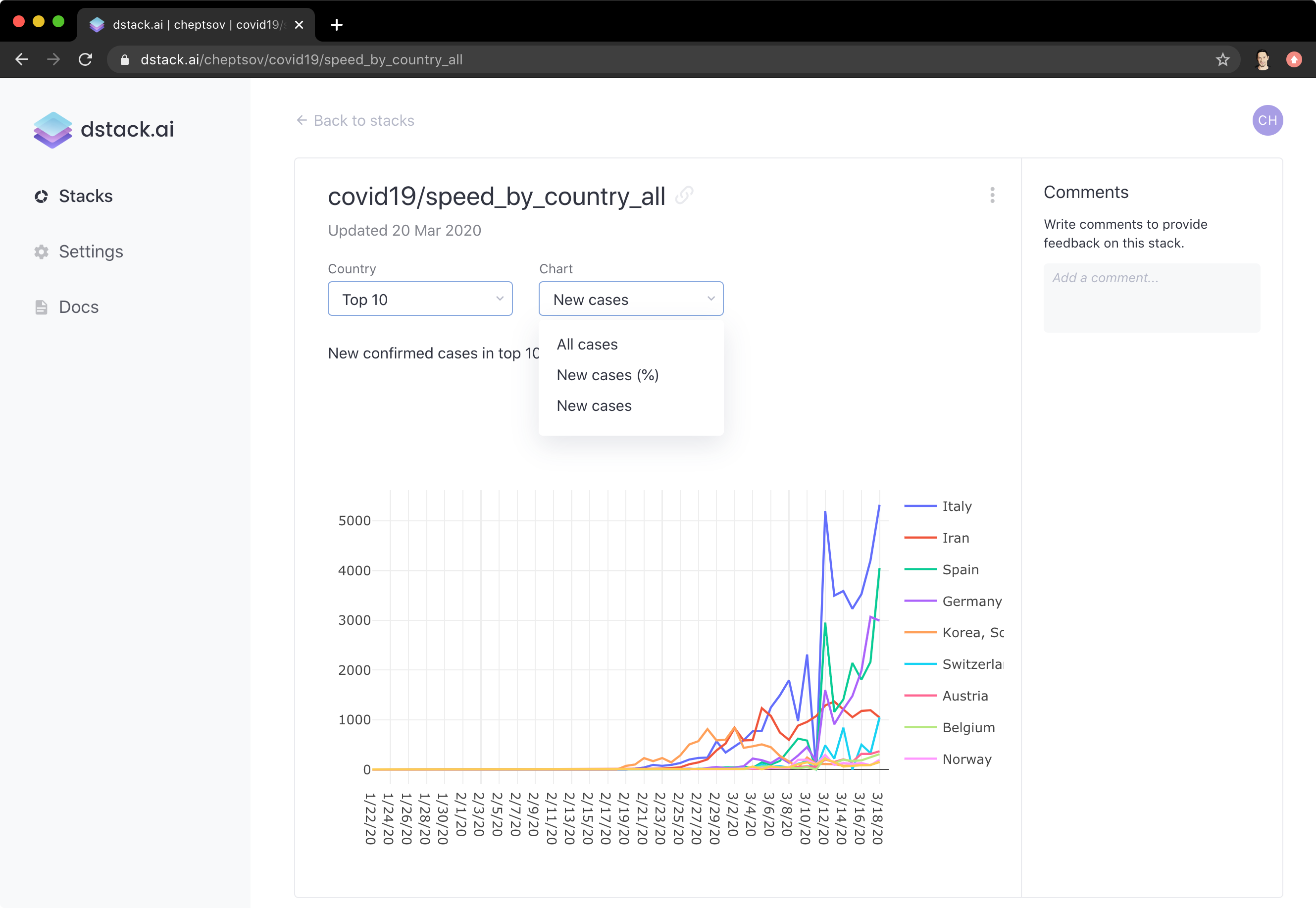That is it for this time. Hope you’ve enjoyed these little exercises and got an idea of how simple actually data analysis is. The source code from this article is available as a Jupyter notebook on GitHub.

Here’s a list of some resources that you can find useful: